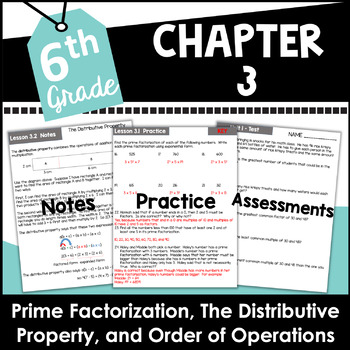# Factors Multiples and Distributive Property 6th Grade Math Unit

Rated 4.25 out of 5, based on 4 reviews
4 Ratings;
5th - 7th
Subjects
Resource Type
Standards
Formats Included
• PDF
Pages
70 pages
\$8.00
List Price:
\$10.00
You Save:
\$2.00
\$8.00
List Price:
\$10.00
You Save:
\$2.00
Report this resource to TPT
##### Also included in
1. Do you teach 6th grade? Are you looking for a new math curriculum? Well, you are in luck. In this 6th grade math NO PREP product you get 8 complete units. This common core aligned product will engage your sixth grade students for an entire year. All you have to do is print these for your student
Price \$88.00Original Price \$129.00Save \$41.00

### Description

In this NO PREP product you get an entire unit that covers: factors, multiples, prime numbers, composite numbers, square numbers, common factors, common multiples, greatest common factors, least common multiples, prime factorizations, the distributive property, and order of operations. All you have to do is print and distribute the notes, worksheets, and assessments to your students.

The bookmarks will help you navigate quickly to where you want to go in the PDF.

This is part of an entire curriculum. Click the link below to view it.

6th Grade Math Curriculum Bundle Common Core Aligned

ALL chapters include:

• Notes

• Practice Pages

Chapter 1 includes:

• Factors and Factor pairs

• Prime, Composite, and Square Numbers

• Multiples

Chapter 2 includes:

• Common Factors

• Common Multiples

• Choosing Between Common Factors and Common Multiples

Chapter 3 includes:

• Prime Factorization

• The Distributive Property

• Order of Operations

• Figuring Out Which Operation to Use (Word Problems)

There is also an assessment (quiz) over Chapters 1 and 2 and a test over the entire Unit! There are study guides for these as well.

The Common Core Standards that are included in this unit are:

6.NS.4, 6.EE.1, 6.EE.2a, 6.EE.2b, 6.EE.2c, 6.EE.3, and 6.EE.4

Total Pages
70 pages
Included
Teaching Duration
N/A
Report this resource to TPT
Reported resources will be reviewed by our team. Report this resource to let us know if this resource violates TPT’s content guidelines.

### Standards

to see state-specific standards (only available in the US).
Find the greatest common factor of two whole numbers less than or equal to 100 and the least common multiple of two whole numbers less than or equal to 12. Use the distributive property to express a sum of two whole numbers 1–100 with a common factor as a multiple of a sum of two whole numbers with no common factor. For example, express 36 + 8 as 4 (9 + 2).
Write and evaluate numerical expressions involving whole-number exponents.
Write expressions that record operations with numbers and with letters standing for numbers. For example, express the calculation “Subtract 𝘺 from 5” as 5 - 𝘺.
Identify parts of an expression using mathematical terms (sum, term, product, factor, quotient, coefficient); view one or more parts of an expression as a single entity. For example, describe the expression 2 (8 + 7) as a product of two factors; view (8 + 7) as both a single entity and a sum of two terms.
Evaluate expressions at specific values of their variables. Include expressions that arise from formulas used in real-world problems. Perform arithmetic operations, including those involving whole-number exponents, in the conventional order when there are no parentheses to specify a particular order (Order of Operations). For example, use the formulas V = s³ and A = 6 s² to find the volume and surface area of a cube with sides of length s = 1/2.